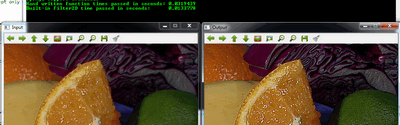OpenCV  3.4.17-dev Open Source Computer Vision

Next Tutorial: Operations with images

Mask operations on matrices are quite simple. The idea is that we recalculate each pixel's value in an image according to a mask matrix (also known as kernel). This mask holds values that will adjust how much influence neighboring pixels (and the current pixel) have on the new pixel value. From a mathematical point of view we make a weighted average, with our specified values.

Our test case

Let's consider the issue of an image contrast enhancement method. Basically we want to apply for every pixel of the image the following formula:

$I(i,j) = 5*I(i,j) - [ I(i-1,j) + I(i+1,j) + I(i,j-1) + I(i,j+1)]$

$\iff I(i,j)*M, \text{where } M = \bordermatrix{ _i\backslash ^j & -1 & 0 & +1 \cr -1 & 0 & -1 & 0 \cr 0 & -1 & 5 & -1 \cr +1 & 0 & -1 & 0 \cr }$

The first notation is by using a formula, while the second is a compacted version of the first by using a mask. You use the mask by putting the center of the mask matrix (in the upper case noted by the zero-zero index) on the pixel you want to calculate and sum up the pixel values multiplied with the overlapped matrix values. It's the same thing, however in case of large matrices the latter notation is a lot easier to look over.

The Basic Method

Now let us see how we can make this happen by using the basic pixel access method or by using the filter2D() function.

Here's a function that will do this:

We create an output image with the same size and the same type as our input. As you can see in the storing section, depending on the number of channels we may have one or more subcolumns.

The filter2D function

Applying such filters are so common in image processing that in OpenCV there is a function that will take care of applying the mask (also called a kernel in some places). For this you first need to define an object that holds the mask:

Then call the filter2D() function specifying the input, the output image and the kernel to use:

The function even has a fifth optional argument to specify the center of the kernel, a sixth for adding an optional value to the filtered pixels before storing them in K and a seventh one for determining what to do in the regions where the operation is undefined (borders).

This function is shorter, less verbose and, because there are some optimizations, it is usually faster than the hand-coded method. For example in my test while the second one took only 13 milliseconds the first took around 31 milliseconds. Quite some difference.

For example: